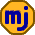## Thursday, May 19, 2022

### Interest on a Car Lease

I’ve written before on how to calculate payments on a car lease.  However, when I began reading Jorge Diaz’s book Car Leasing Done Right, I saw that he believes the interest calculation is different from what I’ve seen everywhere else.

Update 2022-05-19: Jorge Diaz confirmed that his interest calculation was wrong and that he intends to fix it in the next version of his book.

Diaz gives the following example:

MSRP \$27,799 + PDI \$1825 = Vehicle cost of \$29,624
Term: 48 months
Residual Value: \$14,561
Interest Rate: 3.99%
HST: 13%

Diaz calculates the total interest paid over the 4-year lease to be \$1217.01.  This figure is consistent with taking the difference between vehicle cost and the residual value and calculating interest on this as it declines to zero.  We can estimate this by starting with cost minus residual (\$29,624 - \$14,561 = \$15,603).  The average balance owing will be about half of this.  Then we multiply by 4 years and 3.99% to get \$1202.  This is just an estimate, but it comes fairly close to Diaz’s figure.

However, everywhere else I’ve looked says the interest owing on a lease is calculated on the full vehicle cost as it declines down to the residual value.  Estimating once again, the average amount owing would be (\$29,624 + \$14,561)/2.  After multiplying this by 4 years and 3.99% interest, we get \$3526.

Diaz says he got his figure from the “Hyundai Canada Build Tool.”  However, when I looked at this tool, it didn’t give the residual value or the total interest paid, so I couldn’t learn much from it.  But I went to the Canadian Automobile Protection Association (APA) to use their lease calculator.  The result was that the pre-tax interest was \$3521.16, which is close to my estimate and way off Diaz’s figure.  Further, the APA lease calculator gave after-tax lease monthly payments of \$437.50, but Diaz says it is \$377.84.

My conclusion is that lease interest is calculated on the entire vehicle cost as it declines down to the residual value, rather than on just the difference between vehicle cost and residual value as this figure declines to zero.

1.You're showing much more diplomacy than I would have, haha. It makes zero sense to me why a car company would charge interest on only the non-residual portion... After all, they are giving you the entire asset for that time, so that's what you're borrowing and paying interest on. I'd change my view when they start leasing fractions of a car! (I'll need at least three wheels, I suppose).

1.Hi Sebastien,

I agree that it makes sense to charge interest on the entire car. However, it might be possible to tinker with the sale price or residual value to compensate for a nonsensical interest calculation. This is the only way I can make this make any sense (other than Diaz simply being wrong).

2.By that logic, it seems that it would cost the same to lease a brand new vehicle worth \$30,000 that depreciates to \$15,000 as it would a used vehicle worth \$15,000 that depreciates to \$0.

1.An unbeatable argument!